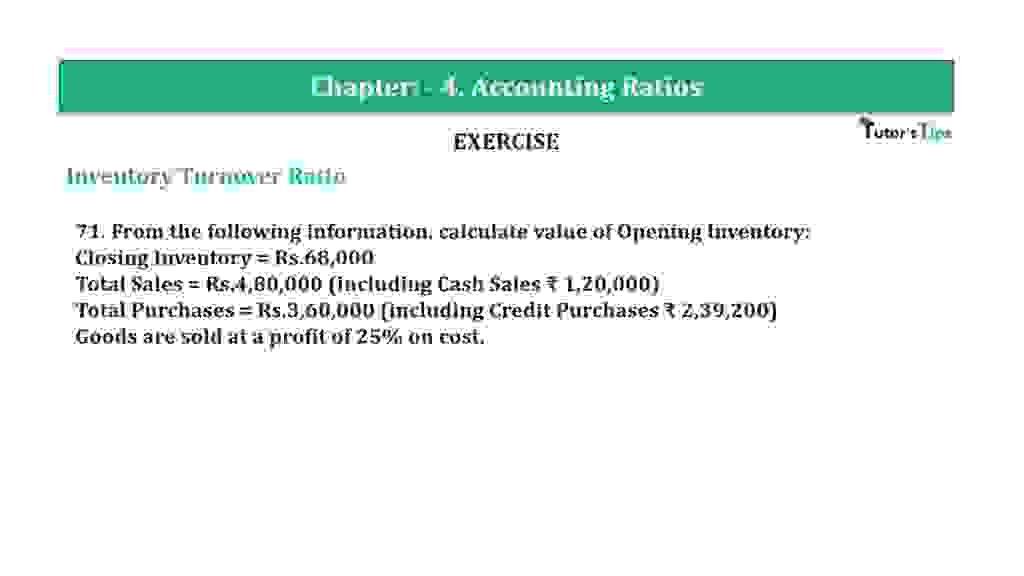# Question 71 Chapter 4 of +2-B – T.S. Grewal 12 ClassQuestion 71 Chapter 4 of +2-B

Interest Coverage Ratio

71. From the following information, calculate the value of the Opening Inventory:
Closing Inventory = Rs.68,000
Total Sales = Rs.4,80,000 (including Cash Sales ₹ 1,20,000)
Total Purchases = Rs.3,60,000 (including Credit Purchases ₹ 2,39,200)
Goods are sold at a profit of 25% on cost.

### The solution of Question 71 Chapter 4 of +2-B: –

 Gross Profit is 25% of Cost. Cost of Goods of Goods Sold = X = Rs. 65,000
 Gross Profit = X x 25 = 25x 100 100

Cost of Goods of Goods Sold = Sales – Gross Profit

 X = Rs.4,80,000 – 25x 100

 125x = Rs.4,80,000 100

 X = Rs. 4,80,000  X 100 125

 Cost of Goods of Goods Sold = Rs. 3,84,000 Cost of Goods of Goods Sold = Opening Inventory + Purchases – Closing Inventory Rs. 3,84,000 = Opening Inventory + Rs. 3,60,000 – Rs. 68,000 Opening Inventory = Rs. 3,84,000 – Rs. 2,92,000 = Rs. 92,000

Balance Sheet: Meaning, Format & Examples

Thanks, Please Like and share with your friends

Comment if you have any question.

Also, Check out the solved question of previous Chapters: –

### T.S. Grewal’s Double Entry Book Keeping (Vol. II: Accounting for Companies)T.S. Grewal’s Analysis of Financial Statements